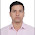## Subsets:

Given a set of distinct integers, S, return all possible subsets.
Note:
• Elements in a subset must be in non-descending order.
• The solution set must not contain duplicate subsets.
For example,
If S = [1,2,3], a solution is:
[
,
,
,
[1,2,3],
[1,3],
[2,3],
[1,2],
[]
]

### Analysis:

The easiest idea is using the binary numbers.
e.g.
set [a,b,c], write the binary numbers of length 3.

000    []
001    [a]
010    [b]
011    [ab]
100    [c]
101    [ac]
110    [bc]
111    [abc]

Then the problem is pretty easy, for each number in binary format,  check which bit is 1 or 0, we just add the ith number into the set if the ith bit in the number is 1.

Bit manipulation is enough for the check, we just have to shift the number 1 one bit left each time, a simple AND operation will give us the bit value.

### Code(C++):

class Solution {
public:
vector<vector<int>> subsets(vector<int>& nums) {
int n = nums.size();
vector< vector<int> > res;
if (n == 0){ return res;}

for (int i=0;i< pow(2,n); i++){
vector<int> tmp;
for (int j=0;j<n;j++){
if ( (i& (1<<j)) > 0 ){
tmp.push_back(nums[j]);
}
}
res.push_back(tmp);
}
return res;
}
};


### Code (Python):

class Solution(object):
def subsets(self, nums):
"""
:type nums: List[int]
:rtype: List[List[int]]
"""
res = []
n = len(nums)
if n==0:
return res

for i in range(pow(2, n)):
l = []
for j in range(n):
if i & (1 << j) > 0:
l.append(nums[j])
res.append(l)
return res



1.A better getBinary program could be as follows:

string getBinary(int d, int len){
stack st;
while(d > 0){
char res = char((d%2)+'0');
st.push(res);
d = d/2;
}
int n = st.size();
for(int i = 0; i < len-n; ++i)
st.push('0');
string str;
while(!st.empty()){
str += st.top();
st.pop();
}
return str;
}

2.This comment has been removed by the author.

3.This comment has been removed by the author.

4.//EASY Solution
http://code.geeksforgeeks.org/eLe0Cw